# CHSL (10+2) Model Papers Mock Test -1 Arithmetic

## CHSL (10+2) Model Papers Mock Test -1 Arithmetic

CHSL (10+2) Model Papers Mock Test -1 Arithmetic

1. A boy was asked to multiply a certain number by 50. He multiplied it by 30 and got his answer less than the correct one by 400. The number to be multiplied was :

(a) 10
(b) 20
(c) 30
(d) 40

2. 0.6 of a number is equal to 0.08 of another number. The ratio of the numbers will be

(a) 3 : 4
(b) 4 : 3
(c) 2 : 15
(d) 2 : 9

3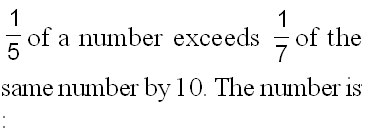(a) 125
(b) 150
(c) 175
(d) 200

4.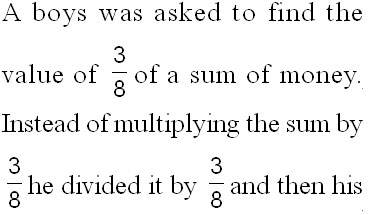(a) Rs. 9
(b) Rs. 24
(c) Rs. 64
(d) Rs. 1,320

5. The sum of three consecutive odd natural numbers each divisible by 3 is 72. What is the largest among them?

(a) 21
(b) 24
(c) 27
(d) 36

6. If *381 is divisible by 11, then the digit at tae place of * is

(a) 0
(b) 1
(c) 4
(d) 7

7. By which smallest number should 5808 be multiplied so that it becomes a perfect square?

(a) 2
(b) 7
(c) 11
(d) 3

8. The least number which when divided by 4, 6, 8, 12 and 16 leaves a remainder of 2 in each case is

(a) 46
(b) 48
(c) 50
(d) 56

9. Sum of two numbers is 40 and their product is 375. What will be the sum of their reciprocals?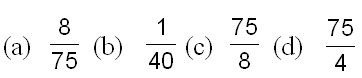10. The product of the LCM and HCF of two numbers is 24. The difference of the two numbers is 2. Find the numbers ?

(a) 8 and 6
(b) 8 and 10
(c) 2 and 4
(d) 6 and 4 3

11.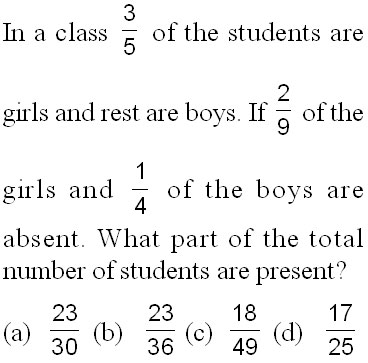12. Two numbers differ by 5. If their product is 336, the sum of the two numbers is

(a) 21
(b) 28
(c) 37
(d) 51

13. The maximum number of students among whom 1001 pens and 910 pencils can be distributed in such a way that each student gets same number of pens and. same number of pencils, is:

(a) 91
(b) 910
(c) 1001
(d) 1911

14. Unit digit in (264)102 + (264)103 is:

(a) 0
(b) 4
(c) 6
(d) 8

15. Which one of the following is the least?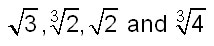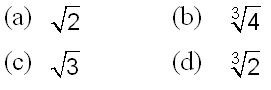16.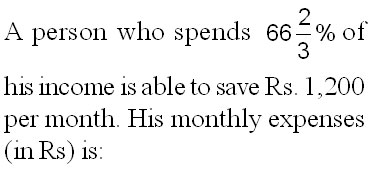(a) 1,200
(b) 2,400
(c) 3,000
(d) 3,200

17. If 80% of A = 50% of B and B = x% of A, then the value of x is:

(a) 400
(b) 300
(c) 160
(d) 150

18. If x is 80% of y, what percent of x is y?

(a) 75%
(b) 80%
(c) 100%
(d) 125%

19. In a town, the population was 8000. In one year, male population increased by 10% and female population increased by 8% but the total population increased by 9%. The number of males in the town was

(a) 4000
(b) 4500
(c) 5000
(d) 6000

20. In an examination, there were 1000 boys and 800 girls. 60% of the boys and 50% of the girls passed. Find the percent of the candidates failed?

(a) 46.4
(b) 48.4
(c) 44.4
(d) 49.6

21. If A exceeds B by 40%, B is less than C by 20%, then A C is,:

(a) 28 : 25
(b) 26 : 25
(c) 3 : 2
(d) 3 : 1

22. Price of sugar rises by 20%. By how much percent should the consumption of sugar be reduced so that the expendi­ture does not change?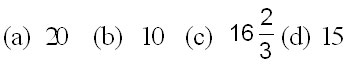23. In a school 70% of the students are girls. The number of boys are 510. Then the total number of students in the school is

(a) 850
(b) 1700
(c) 1830
(d) 1900

24. Applied to a bill for Rs. 1,00,000 the difference between a discount of 40% and two successive discounts of 36% and 4% is

(a) Nil
(b) Rs. 1,440
(c) Rs. 2,500
(d) Rs. 4,000

25. A tradesman marks his goods 10% above his cost price. If he allows his customers 10% discount on the marked price, how much profit or loss does he make, if any?

(a) 1% gain
(b) 1% loss
(c) 5% gain
(d) No gain, no loss

26. A discount of 15% on one article is the same as discount of 20% on a second article. The costs of the two articles can be

(a) Rs. 85, Rs. 60
(b) Rs. 60, Rs. 40
(c) Rs. 40, Rs. 20
(d) Rs. 80, Rs. 60

27. An agent gets a commission of 2.5% on the sales of cloth. If on a certain day, he gets Rs. 12.50 as commission, the cloth sold through him on that day is worth

(a) Rs. 250
(b) Rs. 500
(c) Rs. 750
(d) Rs. 1,250

28. A sum of money doubles itself at compound interest in 15 years. In how many years it will become eight times?

(a) 30
(b) 45
(c) 50
(d) 60

29. Compound interest (compounded annually) on a certain sum of money for 2 years at 4% per annum is Rs. 102. The simple interest on the same sum for the same rate and for the same period will be

(a) Rs. 99
(b) Rs. 101
(c) Rs. 100
(d) Rs. 98

30. A sum of money becomes 6 of itself in 3 years at a certain rate of simple interest. The rate per annum is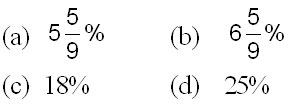31. The simple interest on a certain sum at 5% per annum for 3 years and 4 years differ by Rs. 42. The sum is

(a) Rs. 210
(b) Rs. 280
(c) Rs. 750
(d) Rs. 840

32. Oranges are bought at 7 for Rs. 3. At what rate per hundred must they be sold to gain 33%?

(a) Rs. 56
(b) Rs. 60
(c) Rs. 58
(d) Rs. 57

33. The cost price of 36 books is equal to the selling price of 30 books. The gain is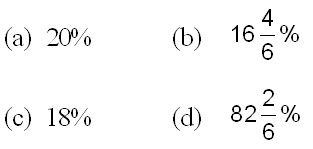34. A man wanted to sell an article with 20% profit; but he actually sold at 20% loss for Rs. 480. At what price he wanted to sell it to earn the profit?

(a) Rs. 720
(b) Rs. 840
(c) Rs. 600
(d) Rs. 750

35. A person sells two machines at Rs. 396 each. On one he gains 10%/0 and on the other he loses 10%. His profit or loss in the whole transaction is

(a) no gain no loss
(b) 1 % loss
(c) 1% profit
(d) 8% profit

36. A trader bought 10 kg of apples for Rs. 405 out of which 1 kg of apples were found to be rotten. If he wishes to make a profit of 10%, at what rate should he sell the remaining apples per kg?

(a) Rs. 45
(b) Rs. 49.50
(c) Rs. 50
(d) Rs. 51

37. If a : b = 7 : 9 and b : c = 15 : 7, then what is a : c?

(a) 5 : 3
(b) 3 : 5
(c) 7 : 21
(d) 7 : 15

38.(a) A, Rs. 28
(b) B, Rs. 3
(c) C, Rs. 20
(d) C, Rs. 25

39. A and B have money in the ratio 2 : 1. If A gives Rs. 2 to B, the money will be in the ratio 1 : 1. What were the initial amounts they had?

(a) Rs. 12 and Rs. 6
(b) Rs. 16 and Rs. 8
(c) Rs. 8 and Rs. 4
(d) Rs. 6 and Rs. 3

40. The incomes of A, B and C are in the ratio 3 : 7 : 4 and their expenses in the ratio 4 : 3 : 5. If A saves Rs. 300 out of an income of Rs. 2,400, the savings of B and C are

(a) Rs. 4.025 and Rs. 575
(b) Rs. 1.575 and Rs 2.625
(c) Rs. 2.750 and Rs. 1.525
(d) Rs. 3.725 and Rs. 1.525

41. Find the measure of an angle which is complement of itself.

(a) 45°
(b) 36°
(c) 42°
(d) 35°

42. An angle is equal to five times its supplement. Find its measure.

(a) 110°
(b) 140°
(c) 150°
(d) 100°

43. The supplement of an angle is one-fourth of itself. Determine the angle and its supplement.

(a) 132°, 48°
(b) 156°, 24°
(c) 118°, 62°
(d) 144°, 36°

44. Two complementary angles differ by 18°. Find the angles.

(a) 42°, 60°
(b) 36°, 54°
(c) 24°, 66°
(d) 18°, 72°

45. If two supplementary angles are in the ratio 1 : 5. Find the difference of the angles.

(a) 64°
(b) 90°
(c) 36°
(d) 70°

46. Find the value of k, if the distance between the points (1, 4), (k, 1) is 5.

(a) 3 or 5
(b) –3 or 5
(c) 3 or –5
(d) None of these

47. The points whose coordinates are (2, 2), (6, 3), (4, 11) forms

(a) a isosceles triangle
(b) a right angle
(c) scalene triangle
(d) an equilateral

48. The following points A (–1, 0), B (3, 1), C (2, 2) and D (–2, 1) taken in order form a

(a) rectangle
(b) parallelogram
(c) square
(d) None of these

49. Which of the following expression is a polynomial?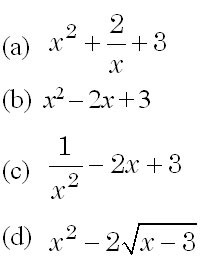50. Which of the following expression is a polynomial in one variable?

(a) 3x2 –2y + 3
(b) –x2 + 6x – 5 = 0
(c) x2 + y2 = –3
(d) 2x2 – 2y-1 = 0